## Stability Matrix

Given a system of two ordinary differential equations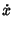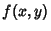(1)(2)

letanddenote Fixed Points with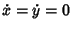, so(3)(4)

Then expand aboutso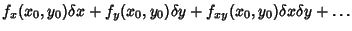(5)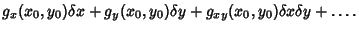(6)

To first-order, this gives(7)

where the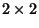Matrix, or its generalization to higher dimension, is called the stability matrix. Analysis of the Eigenvalues (and Eigenvectors) of the stability matrix characterizes the type of Fixed Point.

See also Elliptic Fixed Point (Differential Equations), Fixed Point, Hyperbolic Fixed Point (Differential Equations), Linear Stability, Stable Improper Node, Stable Node, Stable Spiral Point, Stable Star, Unstable Improper Node, Unstable Node, Unstable Spiral Point, Unstable Star

References

Tabor, M. Linear Stability Analysis.'' §1.4 in Chaos and Integrability in Nonlinear Dynamics: An Introduction. New York: Wiley, pp. 20-31, 1989.